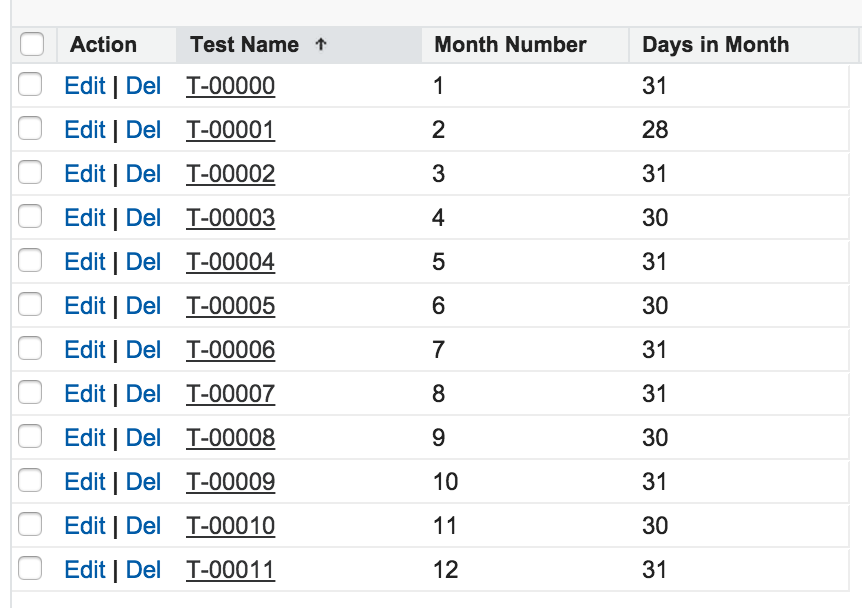# A Simple Salesforce Formula for the Number of Days in a Month

, salesforcedevs, dates, Developer, Force.com, formula, Salesforce, Tips

One of the most common problems that seems to be encountered on the platform is to calculate the number of days in a particular month of the year in order to aid in the setting of an invoice date or other such information - for example you want to set the date of the next invoice to be the end of the next month. There are a lot of ways of doing this involving a lot of if formulas and branches that can cause the size of a formula to be too large or have issues dealing with December or February. When I stumbled across this blog post by Chris McEnroe for calculating the number of days in a month using a mathematical formula, my interest was piqued.

You can read through the information at your own leisure (it is slightly mathematical in nature - some people may find it slightly involved) but I decided to create a simple Salesforce formula that allows you to retrieve the number of days in a month for a particular month. We have a number field here called Month_Number__c which we are using to pass in the month we are working with (you could do this as MONTH(Some_Date_Field__c)) and then our formula is simply returning the number of days for that month. The formula is then

Here is a screenshot of it working for all the months.Note: this formula does not deal with 29 days in a leap year. For most situations this is unlikely to matter.